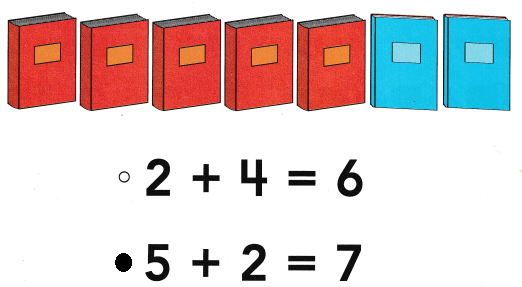# Texas Go Math Kindergarten Lesson 13.2 Answer Key Sums Up to 7

Refer to our Texas Go Math Kindergarten Answer Key Pdf to score good marks in the exams. Test yourself by practicing the problems from Texas Go Math Kindergarten Lesson 13.2 Answer Key Sums Up to 7.

## Texas Go Math Kindergarten Lesson 13.2 Answer Key Sums Up to 7

Essential Question
How can you show and write addition sentences for sums to 7?

Explore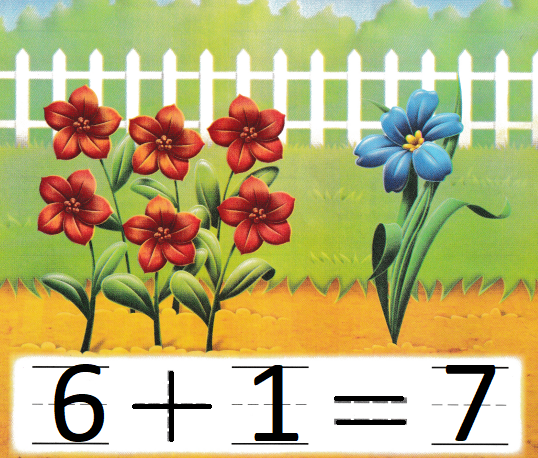Explanation:
6 red flowers and 1 blue flower
6 + 1 = 7
The sum of 6 and 1 is 7

Directions
Listen to the addition word problem. Write the numbers to complete the addition sentence. Trace the symbols.

Share and Show

Question 1.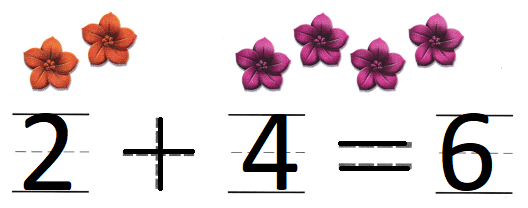Explanation:
2 red flowers and 4 purple flowers
2 + 4 = 6
The sum of 2 and 4 is 6

Question 2.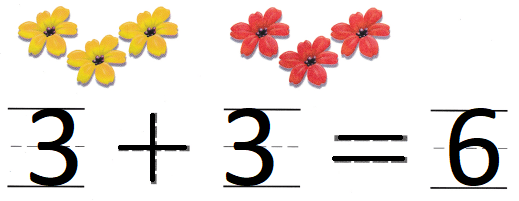Explanation:
3 yellow flowers and 3 red flowers
3 + 3 = 6
The sum of 3 and 3 is 6

Question 3.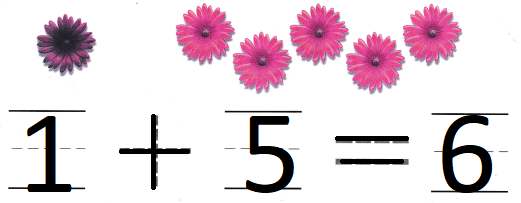Explanation:
1 purple flower and 5 pink flowers
1 + 5 = 6
The sum of 1 and 5 is 6

Directions

Question 4.Explanation:
4 blue flowers and 3 pink flowers
4 + 3 = 7
The sum of 4 and 3 is 7

Question 5.Explanation:
1 purple flower and 6 orange flowers
1 + 6 = 7
The sum of 1 and 6 is 7

Question 6.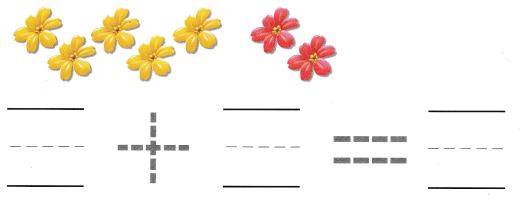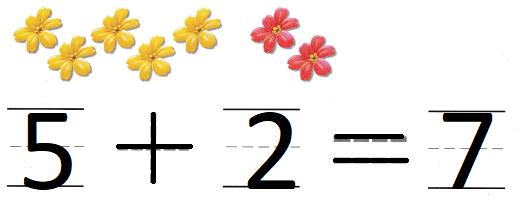Explanation:
5 yellow flowers and 2 red flowers
5 + 2 = 7
the sum of 5 and 2 is 7

Directions

Home Activity

• Have your child use his or her fingers on two hands to show two numbers and tell how many in all.

Problem Solving

Question 7.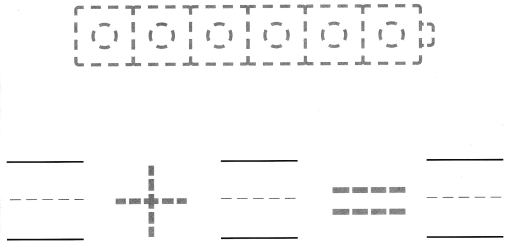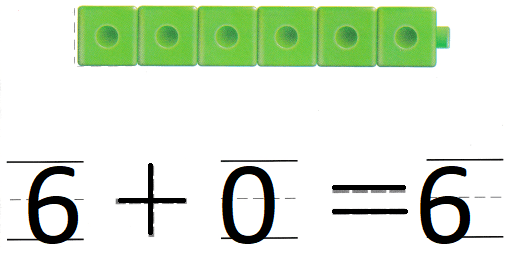Explanation:
Peter and Grant have six flowers in their garden.
Peter has no flowers.
6 + 0 = 6
6 flowers that Grant have

Question 8.Explanation:
Suzie has five red flowers.
She gets one more purple flower.
5 + 1 = 6
The sum of 5 and 1 is 6

Directions
7. Peter and Grant have six flowers in their garden. Peter has no flowers. How many flowers does Grant have? Color the cube train to show the number pair. Complete the addition sentence. 8. Choose the correct answer. Suzie has five red flowers. She gets one more purple flower. What does the picture show?

### Texas Go Math Kindergarten Lesson 13.2 Homework and Practice

Question 1.Explanation:
There are 4 orange leaves on a branch.
There are 2 red leaves on another branch.
4 + 2 = 6
6 leaves are there

Question 2.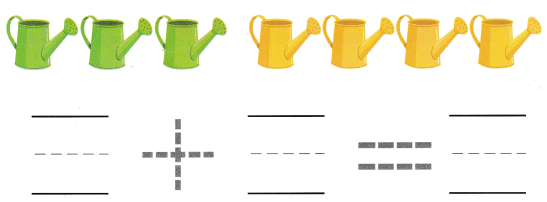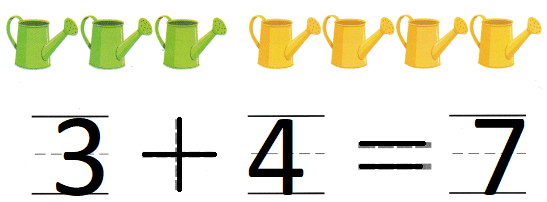Explanation:
The flower nursery has three green watering cans.
They also have four yellow cans.
4 + 3 = 7
7 watering cans are there

Directions
Listen to the addition word problem. Complete the addition sentence. 1. There are 4 orange leaves on a branch. There are 2 red leaves on another branch. How many leaves are there? 2. The flower nursery has three green watering cans. They also have four yellow cans. How many watering cans are there?

Texas Test Prep

Lesson Check

Question 3.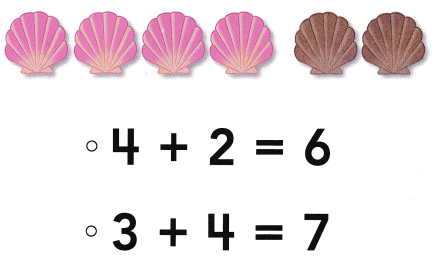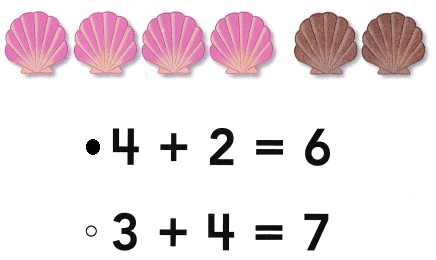Explanation:
Jackie has 4 pink shells.
She gets two brown shells
4 + 2 = 6
so, 6 shells
The sum of 4 and 2 is 6

Question 4.